# BITSAT Mathematics Straight Lines and Pair Of Straight Lines MCQs

Refer to BITSAT Mathematics Straight Lines and Pair Of Straight Lines MCQs provided below. BITSAT Full Syllabus Mathematics MCQs with answers available in Pdf for free download. The MCQ Questions for Full Syllabus Mathematics with answers have been prepared as per the latest syllabus, BITSAT books and examination pattern suggested in Full Syllabus by BITSAT, NCERT and KVS. Multiple Choice Questions for Straight Lines and Pair Of Straight Lines are an important part of exams for Full Syllabus Mathematics and if practiced properly can help you to get higher marks. Refer to more Chapter-wise MCQs for BITSAT Full Syllabus Mathematics and also download more latest study material for all subjects

## MCQ for Full Syllabus Mathematics Straight Lines and Pair Of Straight Lines

Full Syllabus Mathematics students should refer to the following multiple-choice questions with answers for Straight Lines and Pair Of Straight Lines in Full Syllabus. These MCQ questions with answers for Full Syllabus Mathematics will come in exams and help you to score good marks

### Straight Lines and Pair Of Straight Lines MCQ Questions Full Syllabus Mathematics with Answers

#### Question: Given the system of straight lines a(2x + y – 3) + b(3x + 2y – 5) = 0, the line of the system situated farthest from the point (4, –3) has the equation

• a) 4x + 11y – 15 = 0
• b) 7x + y – 8 = 0
• c) 4x + 3y – 7 = 0
• d) 3x – 4y + 1 = 0

Answer: 3x – 4y + 1 = 0

#### Question: The angle between the lines whose intercepts on the axes are a, –b and b, –a respectively , is

• a)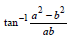• b)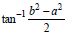• c)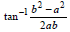• d) None of these#### Question: A ray of light coming from the point (1, 2) is reflected at a point A on the x-axis and then passes through the point (5, 3). The co-ordinates of the point A is

• a)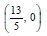• b)• c) (–7, 0)
• d) None of these#### Question: The eq. x2 - 2 √3xy + 3y2 -3x + 3 √3y - 4 = 0 represents

• a) A pair of intersecting lines
• b) a pair of parallel lines with distance between them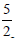• c) A pair of parallel lines with distance between them 5 √2
• d) A conic section, which is not a pair of straight lines

Answer: a pair of parallel lines with distance between them• a) a2
• b) 2a2
• c) a2/2
• d) 3a2

#### Question: The quadratic equation whose roots are the x and y intercepts of the line passing through (1, 1) and making a triangle of area A with the coordinate axes is

• a) x2 + Ax + 2A = 0
• b) x2 - 2Ax + 2A = 0
• c) x2 -Ax + 2A = 0
• d) None of these

Answer: x2 - 2Ax + 2A = 0

#### Question: If 4a2 + b2 + 2c2 + 4ab – 6ac – 3bc = 0, the family of lines ax + by + c = 0 is concurrent at one or the other of the two points-

• a)• b)• c)• d)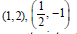#### Question: The nearest point on the line 3x + 4y = 12 from the origin is

• a)• b)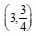• c)• d) None of these• a) 2
• b) 3
• c) 4
• d) 5

#### Question: The family of straight lines (2a + 3b) x + (a – b) y+2a–4b= 0 is concurrent at the point

• a)• b)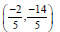• c)• d)• a) (–1, –14)
• b) (3, 4)
• c) (1, 2)
• d) (–4, 13)

#### Question: If the equations of the pairs of opposite sides of a parallelogram are x2 – 5x + 6 = 0 and y2 – 6y + 5 = 0, then equations of its diagonals are

• a) x + 4y = 13, y = 4x – 7
• b) 4x +y = 13, 4y = x – 7
• c) 4x + y = 13, y = 4x – 7
• d) y – 4x = 13, y + 4x = 7

Answer: 4x + y = 13, y = 4x – 7

#### Question: The line 3x – 4y + 7 = 0 is rotated through an angle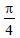in the clockwise direction about the point (–1, 1). The equation of the line in its new position is

• a) 7y + x – 6 = 0
• b) 7y – x – 6 = 0
• c) 7y + x + 6 = 0
• d) 7y – x + 6 = 0

Answer: 7y + x – 6 = 0

• a) 9 : 1
• b) 1 : 9
• c) 3 : 1
• d) √3 : 1

#### Question: The length of the perpendicular from the origin to a line is 7 and line makes an angle of 150° with the positive direction of y-axis the equation of the line is

• a) √3 x + y = 14
• b) √3 x – y = 14
• c) √3 x + y + 14 = 0
• d) √3 x – y + 14 = 0

Answer: √3 x + y = 14

#### Question: The distance between the parallel lines 9x2 – 6xy + y2 +18x - 6y + 8 = 0 is

• a)• b)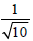• c)• d) None of these• a) √3
• b) √5
• c) √2
• d) 2

• a) 1
• b) 3
• c) 6
• d) 0

• a) 2
• b) 3
• c) 4
• d) 5

#### Question: Given the system of straight lines a(2x + y – 3) + b(3x + 2y – 5) = 0, the line of the system situated farthest from the point (4, –3) has the equation

• a) 4x + 11y – 15 = 0
• b) 7x + y – 8 = 0
• c) 4x + 3y – 7 = 0
• d) 3x – 4y + 1 = 0

Answer: 3x – 4y + 1 = 0

## More Study Material

### BITSAT Full Syllabus Mathematics Straight Lines and Pair Of Straight Lines MCQs

We hope students liked the above MCQs for Straight Lines and Pair Of Straight Lines designed as per the latest syllabus for Full Syllabus Mathematics released by BITSAT. Students of Full Syllabus should download the Multiple Choice Questions and Answers in Pdf format and practice the questions and solutions given in above Full Syllabus Mathematics MCQs Questions on daily basis. All latest MCQs with answers have been developed for Mathematics by referring to the most important and regularly asked topics which the students should learn and practice to get better score in school tests and examinations. Studiestoday is the best portal for Full Syllabus students to get all latest study material free of cost.

### MCQs for Mathematics BITSAT Full Syllabus Straight Lines and Pair Of Straight Lines

Expert teachers of studiestoday have referred to NCERT book for Full Syllabus Mathematics to develop the Mathematics Full Syllabus MCQs. If you download MCQs with answers for the above chapter daily, you will get higher and better marks in Full Syllabus test and exams in the current year as you will be able to have stronger understanding of all concepts. Daily Multiple Choice Questions practice of Mathematics and its study material will help students to have stronger understanding of all concepts and also make them expert on all critical topics. You can easily download and save all MCQs for Full Syllabus Mathematics also from www.studiestoday.com without paying anything in Pdf format. After solving the questions given in the MCQs which have been developed as per latest course books also refer to the NCERT solutions for Full Syllabus Mathematics designed by our teachers

#### Straight Lines and Pair Of Straight Lines MCQs Mathematics BITSAT Full Syllabus

All MCQs given above for Full Syllabus Mathematics have been made as per the latest syllabus and books issued for the current academic year. The students of Full Syllabus can refer to the answers which have been also provided by our teachers for all MCQs of Mathematics so that you are able to solve the questions and then compare your answers with the solutions provided by us. We have also provided lot of MCQ questions for Full Syllabus Mathematics so that you can solve questions relating to all topics given in each chapter. All study material for Full Syllabus Mathematics students have been given on studiestoday.

#### Straight Lines and Pair Of Straight Lines BITSAT Full Syllabus MCQs Mathematics

Regular MCQs practice helps to gain more practice in solving questions to obtain a more comprehensive understanding of Straight Lines and Pair Of Straight Lines concepts. MCQs play an important role in developing understanding of Straight Lines and Pair Of Straight Lines in BITSAT Full Syllabus. Students can download and save or print all the MCQs, printable assignments, practice sheets of the above chapter in Full Syllabus Mathematics in Pdf format from studiestoday. You can print or read them online on your computer or mobile or any other device. After solving these you should also refer to Full Syllabus Mathematics MCQ Test for the same chapter

#### BITSAT MCQs Mathematics Full Syllabus Straight Lines and Pair Of Straight Lines

BITSAT Full Syllabus Mathematics best textbooks have been used for writing the problems given in the above MCQs. If you have tests coming up then you should revise all concepts relating to Straight Lines and Pair Of Straight Lines and then take out print of the above MCQs and attempt all problems. We have also provided a lot of other MCQs for Full Syllabus Mathematics which you can use to further make yourself better in Mathematics

Where can I download latest BITSAT MCQs for Full Syllabus Mathematics Straight Lines and Pair Of Straight Lines

You can download the BITSAT MCQs for Full Syllabus Mathematics Straight Lines and Pair Of Straight Lines for latest session from StudiesToday.com

Can I download the MCQs of Straight Lines and Pair Of Straight Lines Full Syllabus Mathematics in Pdf

Yes, you can click on the links above and download topic wise MCQs Questions PDFs for Straight Lines and Pair Of Straight Lines Full Syllabus for Mathematics

Are the Full Syllabus Mathematics Straight Lines and Pair Of Straight Lines MCQs available for the latest session

Yes, the MCQs issued by BITSAT for Full Syllabus Mathematics Straight Lines and Pair Of Straight Lines have been made available here for latest academic session

How can I download the Straight Lines and Pair Of Straight Lines Full Syllabus Mathematics MCQs

You can easily access the links above and download the Straight Lines and Pair Of Straight Lines Full Syllabus MCQs Mathematics for each topic

Is there any charge for the MCQs with answers for Full Syllabus Mathematics Straight Lines and Pair Of Straight Lines

There is no charge for the MCQs and their answers for Full Syllabus BITSAT Mathematics Straight Lines and Pair Of Straight Lines you can download everything free

How can I improve my MCQs in Full Syllabus Mathematics Straight Lines and Pair Of Straight Lines

Regular revision of MCQs given on studiestoday for Full Syllabus subject Mathematics Straight Lines and Pair Of Straight Lines can help you to score better marks in exams

What are MCQs for Full Syllabus Mathematics Straight Lines and Pair Of Straight Lines

Multiple Choice Questions (MCQs) for Straight Lines and Pair Of Straight Lines Full Syllabus Mathematics are objective-based questions which provide multiple answer options, and students are required to choose the correct answer from the given choices.